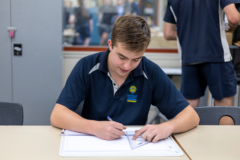## MATHEMATICS DOMAIN#### General Mathematics 1-2• Incorporates a practical approach that equips students with essential skills as future citizens
• Learn statistics, linear graphs, financial modelling and more
• Suits students who enjoy non-algebraic mathematics

Subject Code: V1MGM
Year Level: 11

##### Unit 1 & 2

This unit would benefit a broad range of students. You learn statistics, linear modelling, linear graphs, matrices, recursion and financial modelling.

##### Assessment
• Topic tests
• Assignments
• End of semester exam
##### What sort of student would like General Mathematics?

Someone who:

• Would like to continue with Mathematics with the option of studying Further Mathematics in Year 12
• Requires a maths unit for tertiary study but not to the level of Mathematical Methods
• Is not looking to go on to tertiary studies but may require Mathematics at Year 11 level to undertake a particular pathway.
##### Pre-Requisites

Pathways (Year 10) subjects: Pre-General or Pre-Methods.

##### Other consideration

You need to enjoy Mathematics, in particular the non-algebraic type of mathematics (i.e. Data and Statistics, Financial Mathematics, Networks, Geometry and Trigonometry.)

You must have a Ti-nspire CAS calculator. It is used extensively throughout the unit.

#### Mathematical Methods 1-2• Study of a variety of functions including; Linear, Quadratics and Cubics
• Can lead to degrees in engineering, sciences, economics, commerce and business
• Must be willing to dedicate time to study outside of class

Subject Code: V1MMM
Year Level: 11

##### Unit 1 & 2

Mathematical Methods Unit 1 and 2 is designed as preparation for Mathematical Methods Unit 3 and 4. You begin the year with formal study of a variety of functions including; Linear, Quadratics, Cubics, Quartics, Logarithms and Exponentials. The definitions, algebra and graphical representation of these are studied. Second semester is devoted to Circular Functions, Rates of Change, Calculus, Probability and Statistics. You need to have a Ti-nspire CAS calculator to facilitate their learning and help solve problems.

##### Assessment
• Topic tests
• Technology inclusive exam
• Technology exclusive exam
##### What sort of student would like Mathematical Methods Unit 1 & 2?

Someone who:

• Has a strong interest in Mathematics and enjoys the challenge that an analysis question can provide
• Has a good work ethic – the typical Methods Unit 1 and 2 student completes three to four hours of Methods homework per week
• Is competent at maths.
##### Pre-Requisites

Completion of Pre-Methods or equivalent with a very good understanding of the topics studied. It is not recommended for Year 9 students to accelerate into Methods 1 and 2 unless a formal discussion has taken place with the Mathematics Leader, Steven Ramage.

#### Specialist Mathematics 1-2• For students who wish to undertake an in-depth study of mathematics
• Emphasis on concepts, skills and processes related to mathematical structure, modelling, problem solving and reasoning
• Considering a career in engineering? This subject is recommended for you

Subject Code: V1MSM
Year Level: 11

##### Unit 1 & 2

This unit is also the suggested pre-requisite for the study of Specialist Mathematics Unit 3 and 4.

This unit provides an excellent preparation for Mathematical Methods Unit 3 and 4, particularly for those students who feel they could benefit from an extra year of study before proceeding to the next level of Mathematics. You need to have a CAS Ti-nspire calculator to facilitate learning and help to solve problems.

##### What does this mean for me?

You study a wide range of mathematical units including Algebra, Number Theory, Kinematics, Statistics, Dynamics and Calculus, Trigonometry, Complex Numbers, Graphs of Linear and Non-Linear Functions and Discrete Mathematics.

##### Assessment (each semester)
• Topic tests
• Assignments
• Completion of chapter exercises
• Technology inclusive exam
• Technology exclusive exam
##### What sort of student would like Specialist Mathematics Unit 1 & 2?

Someone who:

• Loves Mathematics, especially Algebra. Some will undertake this as a second Maths
• Is highly organised. This unit requires you to apply yourself consistently and complete all set tasks. Mathematics homework is required after each lesson. It is essential that you keep up to date with the set work
• This unit is very beneficial to the study of Mathematical Methods when studied concurrently with Methods Unit 1 and 2, or Methods Unit 3 and 4.
##### Pre-Requisites

10 Pre-Methods and or Mathematical Methods Unit 1 and 2.